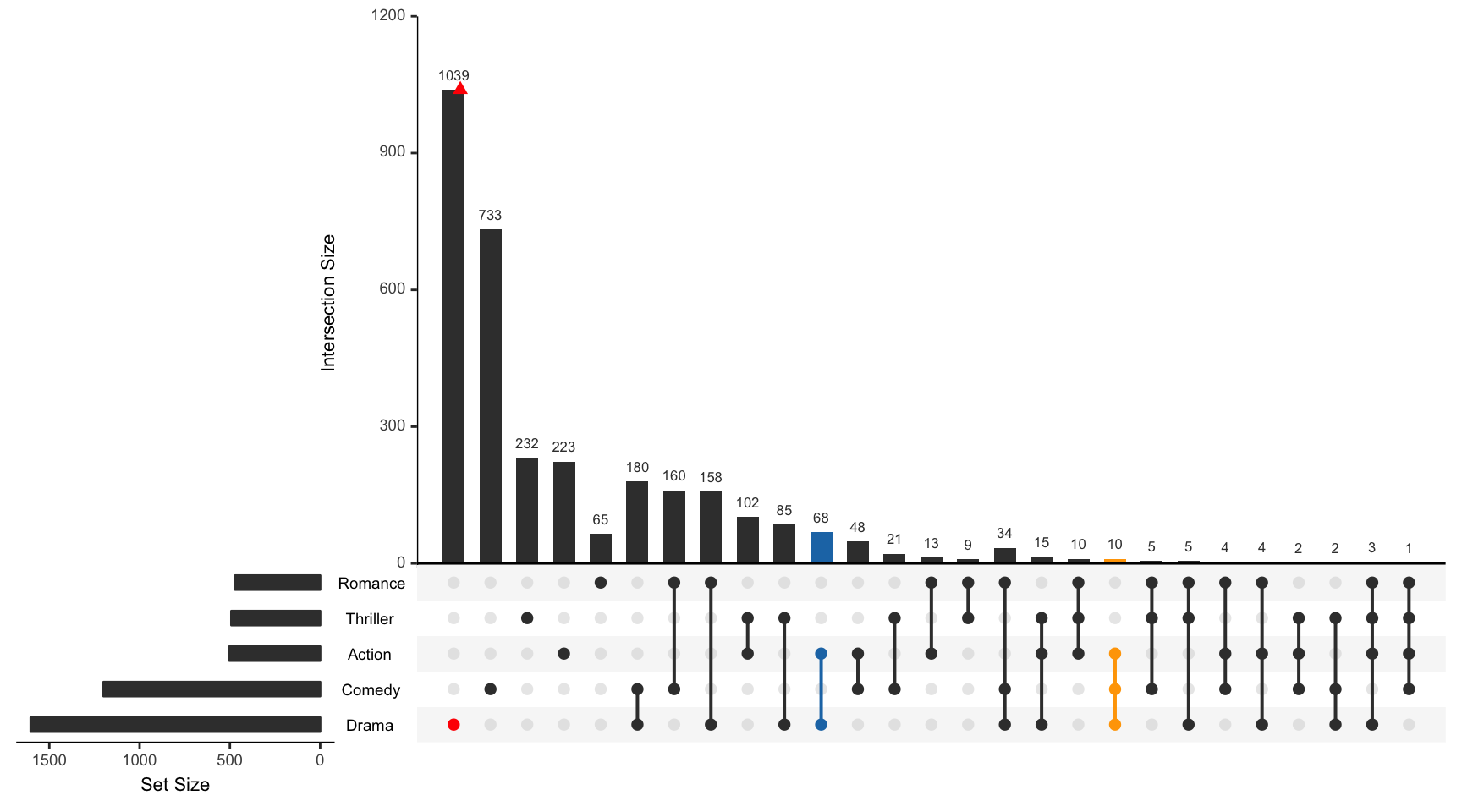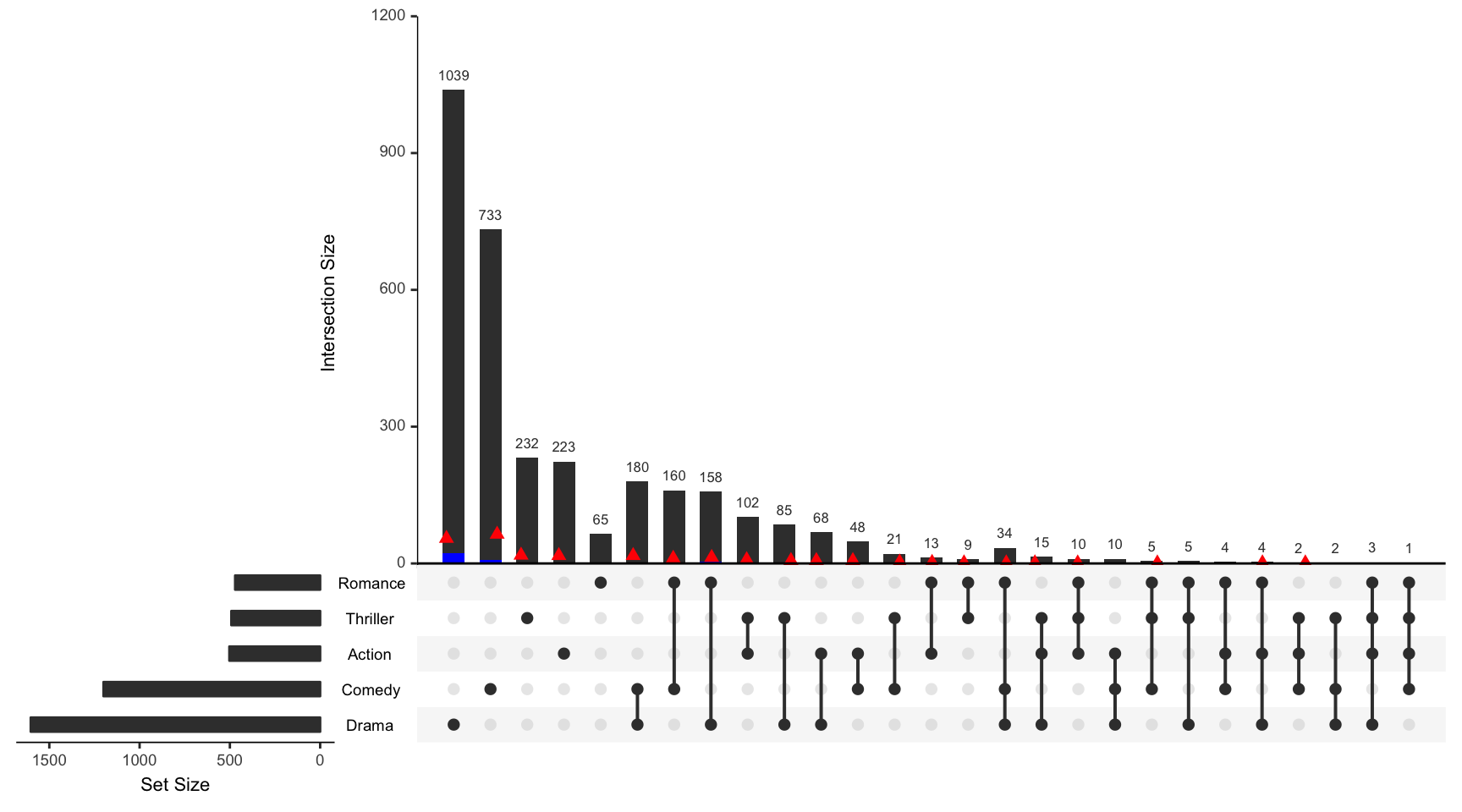For all examples the movies data set contained in the package will be used.

``````library(UpSetR)
movies <- read.csv(system.file("extdata", "movies.csv", package = "UpSetR"),
header = T, sep = ";")``````

## queries Parameter Breakdown

Each list contained in the `queries` parameter takes 4 fields: `query`, `params`, `color`, and `active`.

• `query` specifies which query is going to be run

• `params` is a list of paramters for the query to work on

• `color` is the color that will represent the query on the plot. If no color is provided, a color will be selected from the UpSetR default color palette.

• `active` determines how the query will be represented on the plot. If `active` is `TRUE`, the intersection size bar will be overlayed by a bar representing the query. If `active` is `FALSE`, a jitter point will be placed on the intersection size bar.

To learn how queries can be explored and visualized on an element level see the Attribute Plots vignette.

## Example 1: Built-in Intersection Query

This example shows how to use the built in intersection query, `intersects`, to find or display elements in specific intersections. In this example the color selected for the active query is from the default color palette.

``````upset(movies, queries = list(list(query = intersects, params = list("Drama",
"Comedy", "Action"), color = "orange", active = T), list(query = intersects,
params = list("Drama"), color = "red", active = F), list(query = intersects,
params = list("Action", "Drama"), active = T)))``````## Example 2: Built-In Elements Query

This example shows how to use the built in element query, `elements`, to visualize how certain elements are distributed amongst the intersections.

``````upset(movies, queries = list(list(query = elements, params = list("AvgRating",
3.5, 4.1), color = "blue", active = T), list(query = elements, params = list("ReleaseDate",
1980, 1990, 2000), color = "red", active = F)))``````## Example 3: Using Expression Parameter to Subset Intersection and Element Queries

This example shows how to use the `expression` parameter to subset the results of element and intersection queries.

``````upset(movies, queries = list(list(query = intersects, params = list("Action",
"Drama"), active = T), list(query = elements, params = list("ReleaseDate",
1980, 1990, 2000), color = "red", active = F)), expression = "AvgRating > 3 & Watches > 100")``````